# How To Code RNN And LSTM Neural Networks In Python

In :
import matplotlib.pyplot as plt
import numpy as np
import pandas as pd
import tensorflow as tf
from tensorflow import keras
from tensorflow.keras import layers

tf.__version__

Out:
'2.3.1'

Pandas

### What's so special about text?

Text is categorized as Sequential data: a document is a sequence of sentences, each sentence is a sequence of words, and each word is a sequence of characters. What is so special about text is that the next word in a sentence depends on:

1. Context: which can extend long distances before and after the word, aka long term dependancy.
2. Intent: different words can fit in the same contexts depending on the author's intent.

### What do we need?

We need a neural network that models sequences. Specifically, given a sequence of words, we want to model the next word, then the next word, then the next word, ... and so on. That could be on a sentence, word, or character level. Our goal can be to just make a model to predict/generate the next word, like in unsupervised word embeddings. Alternatively, we could just map patterns in the text to associated labels, like in text classifications. In this notebook, we will be focusing on the latter. However, the networks used for either are pretty similar. The role of the network is most important in processing the textual input, extracting, and modelling the linguistic features. What we then do with these features is another story.

## Recurrent Neural Networks (RNNs)

A Recurrent Neural Network (RNN) has a temporal dimension. In other words, the prediction of the first run of the network is fed as an input to the network in the next run. This beautifully reflects the nature of textual sequences: starting with the word "I" the network would expect to see "am", or "went", "go" ...etc. But then when we observe the next word, which let us say, is "am", the network tries to predict what comes after "I am", and so on. So yeah, it is a generative model!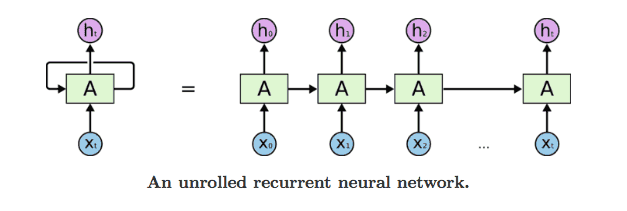### Reber Grammar Classification

Let's start by a simple grammar classification. We assume there is a linguistic rule that characters are generated according to. This is a simple simulation of grammar in our natural language: you can say "I am" but not "I are". More onto Reber Grammar > here.

#### Defining the grammar

Consider the following Reber Grammar:

Reber Grammar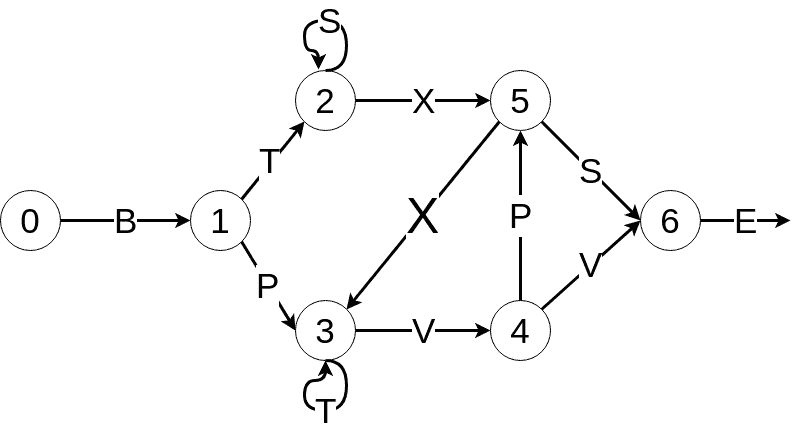Let's represent it first in Python:

In :
default_reber_grammar=[
[("B",1)],  #(state 0) =B=> (state 1)
[("T", 2),("P", 3)],  # (state 1) =T=> (state 2) or =P=> (state 3)
[("X", 5), ("S", 2)], # (state 2) =X=> (state 5) or =S=> (state 2)
[("T", 3), ("V", 4)], # (state 3) =T=> (state 3) or =V=> (state 4)
[("V", 6), ("P", 5)], # (state 4) =V=> (state 6) or =P=> (state 5)
[("X",3), ("S", 6)],  # (state 5) =X=> (state 3) or =S=> (state 6)
[("E", None)]         # (state 6) =E=> <EOS>

]


Let's take this a step further, and use Embedded Reber Grammar, which simulates slightly more complicated linguistic rules, such as phrases!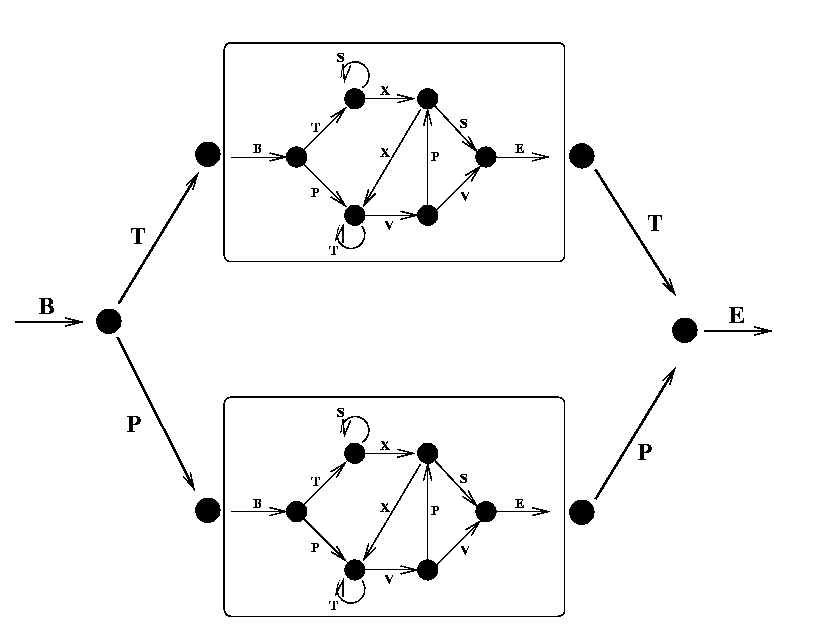In :
embedded_reber_grammar=[
[("B",1)],  #(state 0) =B=> (state 1)
[("T", 2),("P", 3)],  # (state 1) =T=> (state 2) or =P=> (state 3)
[(default_reber_grammar,4)], # (state 2) =REBER=> (state 4)
[(default_reber_grammar,5)], # (state 3) =REBER=> (state 5)
[("P", 6)], # (state 4) =P=> (state 6)
[("T",6)],  # (state 5) =T=> (state 3)
[("E", None)]         # (state 6) =E=> <EOS>

]


Now let's generate some data using these grammars:

#### Generating data

In :
def generate_valid_string(grammar):
state = 0
output = []
while state is not None:
char, state = grammar[state][np.random.randint(len(grammar[state]))]
if isinstance(char, list):  # embedded reber
char = generate_valid_string(char)
output.append(char)
return "".join(output)

In :
def generate_corrupted_string(grammar, chars='BTSXPVE'):
'''Substitute one character to violate the grammar'''
good_string = generate_valid_string(grammar)
idx = np.random.randint(len(good_string))
good_char = good_string[idx]


Let's define all the possible characters used in the grammar.

In :
chars='BTSXPVE'
chars_dict = {a:i for i,a in enumerate(chars)}
chars_dict

Out:
{'B': 0, 'T': 1, 'S': 2, 'X': 3, 'P': 4, 'V': 5, 'E': 6}

One hot encoding is used to represent each character with a vector so that all vectors are equally far away from each other. For example,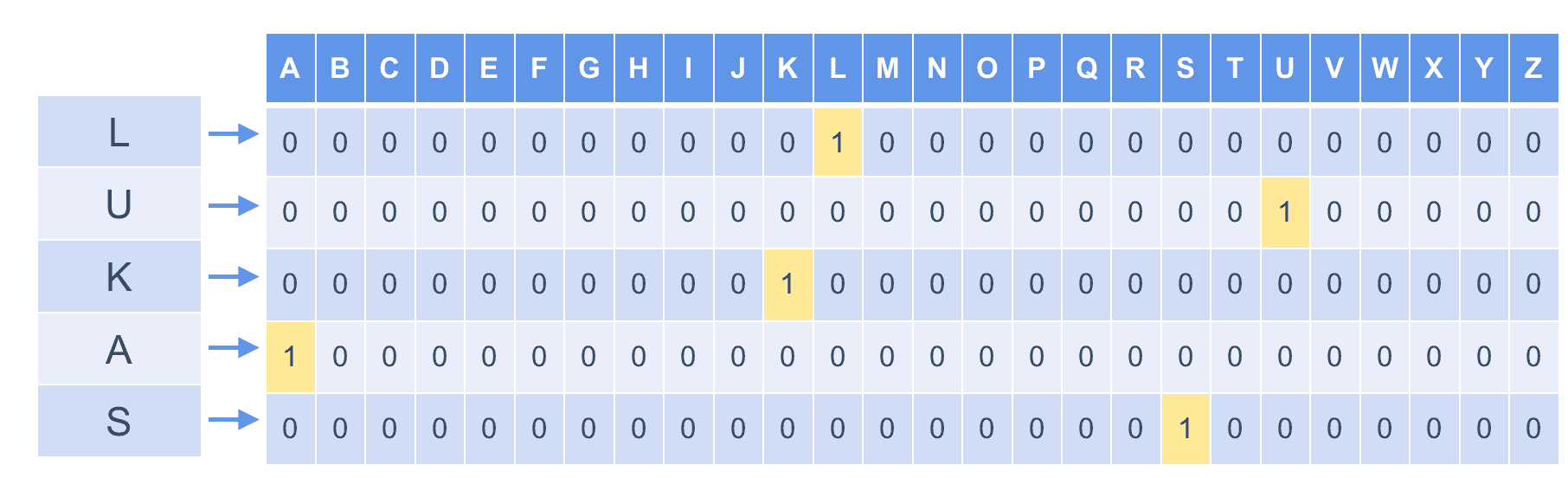In :
def str2onehot(string, num_steps=12, chars_dict=chars_dict):
res = np.zeros((num_steps, len(chars_dict)))
for i in range(min(len(string), num_steps)):
c = string[i]
res[i][chars_dict[c]] = 1
return res


Now let's generate a dataset of valid and corrupted strings

In :
def generate_data(data_size=10000, grammar=embedded_reber_grammar, num_steps=None):
good = [generate_valid_string(grammar) for _ in range(data_size//2)]
bad = [generate_corrupted_string(grammar) for _ in range(data_size//2)]
if num_steps is None:
num_steps = max([len(s) for s in all_strings])
X = np.array([str2onehot(s) for s in all_strings])
l = np.array([len(s) for s in all_strings])
idx = np.random.permutation(data_size)
return X[idx], l[idx], y[idx]

In :
np.random.seed(42)
X_train, seq_lens_train, y_train = generate_data(10000)
X_val, seq_lens_val, y_val = generate_data(5000)
X_train.shape, X_val.shape

Out:
((10000, 12, 7), (5000, 12, 7))

We have 10,000 words, each with 12 characters, and maximum of 7 unique letters (i.e. BTSXPVE)

#### Building the model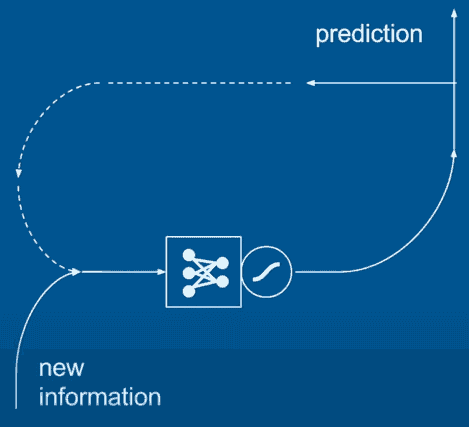source

In :
x = layers.Input(shape=(12, 7)) # we define our input's shape
# first we define our RNN cells to use in the RNN model
# let's keep the model simple ...
cell = layers.SimpleRNNCell(4, activation='tanh')  # ... by just using 4 units (like 4 units in hidden layers)
rnn = layers.RNN(cell)
rnn_output = rnn(x)


We use tanh activation function to make the prediction between -1 and 1 the resulting activation between -1 and 1 is then weighted to finally give us the features to use in making our predictions

We finally add a fully connected layer to map our rnn outputs to the 0-1 classification output. We use a sigmoid function to map the prediction to probabilities between 0 and 1.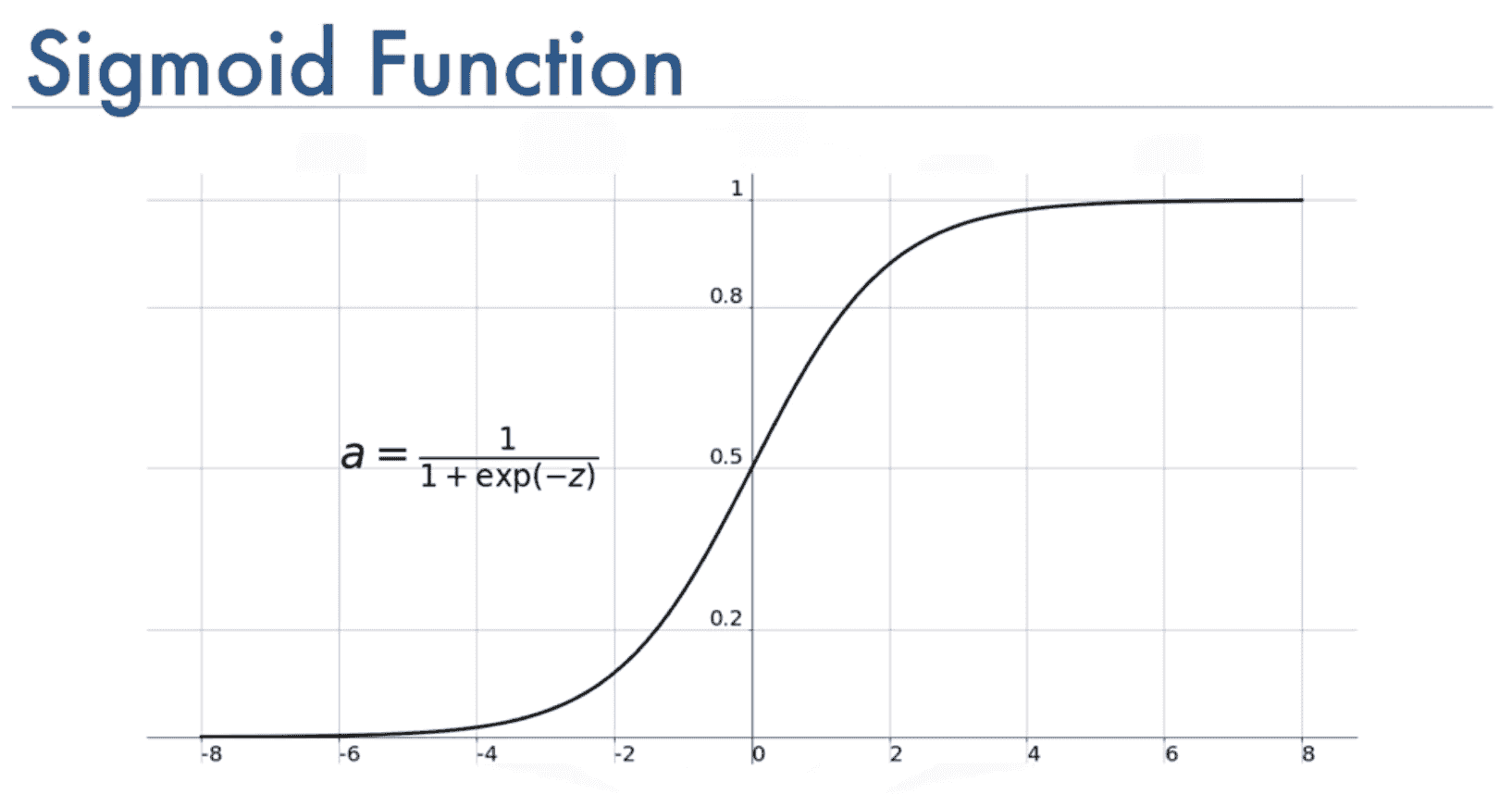In :
output = layers.Dense(units=1, activation='sigmoid')(rnn_output)

In :
# let's compile the model
model = keras.Model(inputs=x, outputs=output)
# loss is binary cropss entropy since this is a binary classification task
# and evaluation metric as f1
model.compile(loss="binary_crossentropy", metrics=["accuracy"])
model.summary()

Model: "functional_3"
_________________________________________________________________
Layer (type)                 Output Shape              Param #
=================================================================
input_2 (InputLayer)         [(None, 12, 7)]           0
_________________________________________________________________
rnn_1 (RNN)                  (None, 4)                 48
_________________________________________________________________
dense_1 (Dense)              (None, 1)                 5
=================================================================
Total params: 53
Trainable params: 53
Non-trainable params: 0
_________________________________________________________________


We have 12 characters in each input, and 4 units per RNN cell, so we have a total of 12x4=48 parameters to learn + 5 more parameters from the fully connected (FC) layer.

In :
# we train the model for 100 epochs
H = model.fit(X_train, y_train, epochs=100, verbose=2, validation_data=(X_val, y_val))

In :
def plot_results(H):
results = pd.DataFrame({"Train Loss": H.history['loss'], "Validation Loss": H.history['val_loss'],
"Train Accuracy": H.history['accuracy'], "Validation Accuracy": H.history['val_accuracy']
})
fig, ax = plt.subplots(nrows=2, figsize=(16, 9))
results[["Train Loss", "Validation Loss"]].plot(ax=ax)
results[["Train Accuracy", "Validation Accuracy"]].plot(ax=ax)
ax.set_xlabel("Epoch")
ax.set_xlabel("Epoch")
plt.show()

In :
plot_results(H)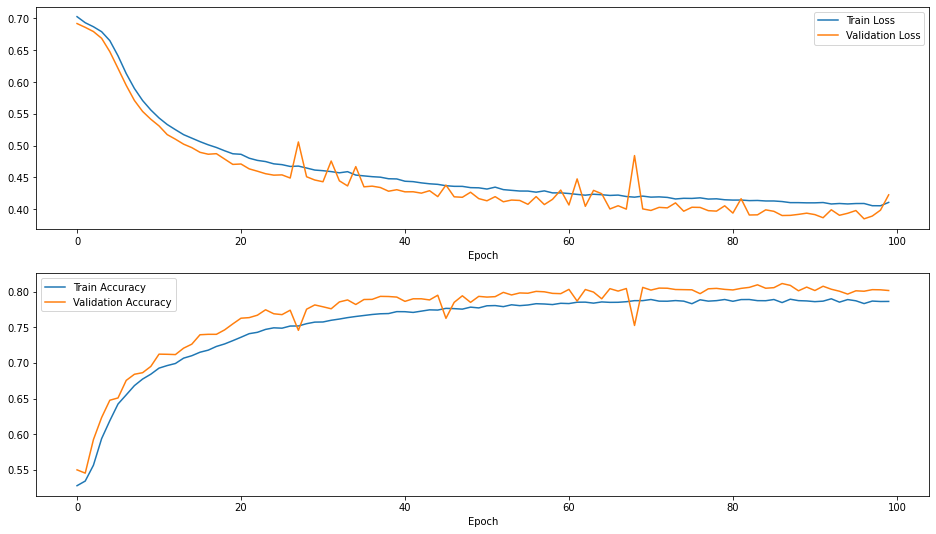## LSTM

Long short-term memory employs logic gates to control multiple RNNs, each is trained for a specific task. LSTMs allow the model to memorize long-term dependancies and forget less likely predictions. For example, if the training data had "John saw Sarah" and "Sarah saw John", when the model is given "John saw", the word "saw" can predict "Sarah" and "John" as they have been seen just after "saw". LSTM allows the model to recognize that "John saw" is going to undermine the possibility for "John", so we won't get "John saw John". Also we won't get "John saw John saw John saw John ..." as the model can predict that what comes after the word after saw, is the end of the sentence.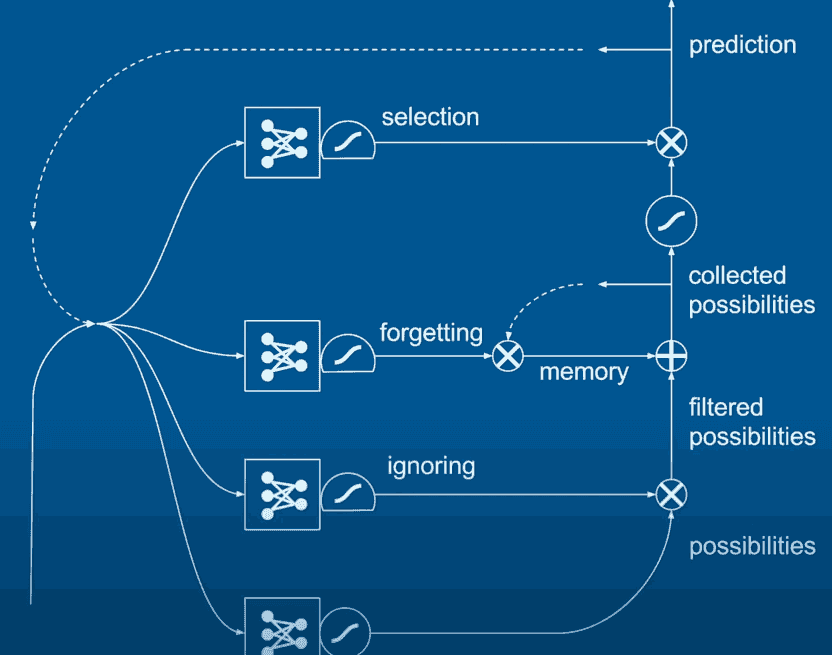source

Now we will apply bidirectional LSTM (that looks both backward and forward in the sentence) for text classification.

### Sentiment Analysis: IMDB reviews

source

NEVER train two models on the same kernel session. We already trained the reber grammar one, so we need to restart the kernel first.

In :
!pip install -q tensorflow_datasets

In :
import tensorflow_datasets as tfds

In :
dataset, info = tfds.load('imdb_reviews', with_info=True,
as_supervised=True)
train_dataset, test_dataset = dataset['train'], dataset['test']


#### Processing the data

1. (optional) take a small sample of the data, since this is just a demo!
2. Align the reviews with their labels
3. Shuffle the data
In :
train = train_dataset.take(4000)
test = test_dataset.take(1000)

In :
# to shuffle the data ...
BUFFER_SIZE = 4000 # we will put all the data into this big buffer, and sample randomly from the buffer
BATCH_SIZE = 128  # we will read 128 reviews at a time

train = train.shuffle(BUFFER_SIZE).batch(BATCH_SIZE)
test = test.batch(BATCH_SIZE)


prefetch: to allow the later elements to be prepared while the current elements are being processed.

In :
train = train.prefetch(BUFFER_SIZE)
test = test.prefetch(BUFFER_SIZE)


#### Text Encoding

Each word in the sentence is going to be replaced with its corresponding index in the vocabulary.

In :
VOCAB_SIZE=1000 # assuming our vocabulary is just 1000 words

encoder = layers.experimental.preprocessing.TextVectorization(max_tokens=VOCAB_SIZE)

encoder.adapt(train.map(lambda text, label: text)) # we just encode the text, not the labels

In :
# here are the first 20 words in our 1000-word vocabulary
vocab = np.array(encoder.get_vocabulary())
vocab[:20]

Out:
array(['', '[UNK]', 'the', 'and', 'a', 'of', 'to', 'is', 'in', 'i', 'it',
'this', 'that', 'br', 'was', 'as', 'with', 'for', 'but', 'movie'],
dtype='<U14')
In :
example, label = list(train.take(1)) # that's one batch
len(example)

Out:
128
In :
example.numpy()

Out:
b'There have been so many many films based on the same theme. single cute girl needs handsome boy to impress ex, pays him and then (guess what?) she falls in love with him, there\'s a bit of fumbling followed by a row before everyone makes up before the happy ending......this has been done many times.<br /><br />The thing is I knew this before starting to watch. But, despite this, I was still looking forward to it. In the right hands, with a good cast and a bright script it can still be a pleasant way to pass a couple of hours.<br /><br />this was none of these.<br /><br />this was dire.<br /><br />A female lead lacking in charm or wit who totally failed to light even the slightest spark in me. I truly did not care if she "got her man" or remained single and unhappy.<br /><br />A male lead who, after a few of his endless words of wisdom, i wanted to kill. Just to remove that smug look. i had no idea that leading a life of a male whore was the path to all-seeing all-knowing enlightenment.<br /><br />A totally unrealistic film filled with unrealistic characters. none of them seemed to have jobs, all of them had more money than sense, a bridegroom who still goes ahead with his wedding after learning that his bride slept with his best friend....plus "i would miss you even if we had never met"!!!!! i could go on but i have just realised that i am wasting even more time on this dross.....I could rant about introducing a character just to have a very cheap laugh at the name "woody" but in truth that was the only remotely humorous thing that happened in the film.'
In :
encoded_example = encoder(example[:1]).numpy()
encoded_example

Out:
array([[ 49,  26,  78,  36, 107, 107,  92, 417,  21,   2, 165, 810, 593,
988, 241, 795,   1, 429,   6,   1,   1,   1,  90,   3,  91, 495,
48,  56, 646,   8, 113,  16,  90, 222,   4, 197,   5,   1,   1,
33,   4,   1, 157, 336, 151,  57, 157,   2, 659,   1,  46,  78,
218, 107,   1,  13,   2, 144,   7,   9, 782,  11, 157,   1,   6,
104,  18, 475,  11,   9,  14, 122, 289, 971,   6,  10,   8,   2,
212, 946,  16,   4,  50, 185,   3,   4,   1, 227,  10,  69, 122,
28,   4,   1,  97,   6,   1,   4, 367,   5,   1,  13,  11,  14,
683,   5,   1,  13,  11,  14,   1,  13,   4, 634, 480,   1,   8,
1,  42,   1,  37, 432, 901,   6, 752,  55,   2,   1,   1,   8,
70,   9, 347, 118,  22, 425,  43,  56, 175,  40, 121,  42,   1,
593,   3,   1,  13,   4,   1, 480,  37, 101,   4, 178,   5,  23,
1, 609,   5,   1,   9, 449,   6, 485,  41,   6,   1,  12,   1,
158,   9,  63,  58, 326,  12, 813,   4, 115,   5,   4,   1,   1,
14,   2,   1,   6,   1,   1,   1,  13,   4, 432,   1,  20,   1,
16,   1, 103, 683,   5,  95, 463,   6,  26,   1,  32,   5,  95,
63,  51, 270,  71, 275,   4,   1,  37, 122, 278,   1,  16,  23,
1, 101,   1,  12,  23,   1,   1,  16,  23, 108,   1,   9,  60,
731,  25,  55,  43,  73,  63, 114,   1,   9,  96, 131,  21,  18,
9,  26,  41,   1,  12,   9, 214,   1,  55,  51,  59,  21,  11,
1,  96,   1,  45,   1,   4, 109,  41,   6,  26,   4,  52, 831,
500,  31,   2, 391,   1,  18,   8, 883,  12,  14,   2,  64,   1,
1, 144,  12, 571,   8,   2,  20]])

#### Creating the model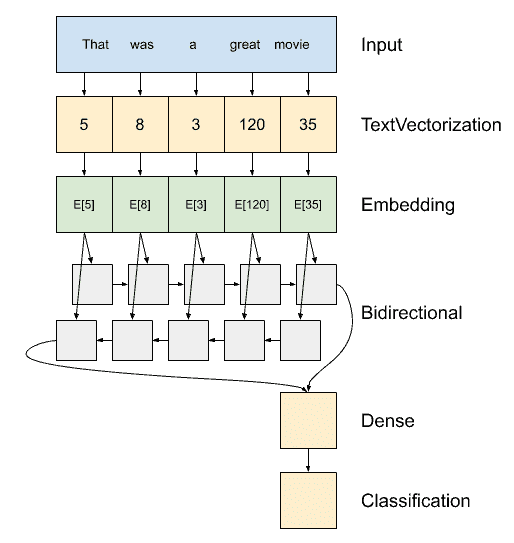In :
model = tf.keras.Sequential([
encoder, # the encoder
tf.keras.layers.Embedding(
input_dim=len(encoder.get_vocabulary()),
output_dim=64,
# Use masking to handle the variable sequence lengths
tf.keras.layers.Bidirectional(layers.LSTM(64)), # making LSTM bidirectional
tf.keras.layers.Dense(32, activation='relu'), # FC layer for the classification part
tf.keras.layers.Dense(1) # final FC layer

])


Let's try it out!

In :
sample_text = ('The movie was cool. The animation and the graphics '
'were out of this world. I would recommend this movie.')
predictions = model.predict(np.array([sample_text]))
print(predictions)

[-0.00052149]


yeah yeah, we haven't trained the model yet.

#### Compiling & training the model

In :
# we will use binary cross entropy again because this is a binary classification task (positive or negative)
# we also did not apply a sigmoid activation function at the last FC layer, so we specify that the
# are calculating the cross entropy from logits
model.compile(
loss=tf.keras.losses.BinaryCrossentropy(from_logits=True),
# adam optimizer is more efficient (not always the most accurate though)
metrics=['accuracy']
)

In :
model.summary()

Model: "sequential"
_________________________________________________________________
Layer (type)                 Output Shape              Param #
=================================================================
text_vectorization (TextVect (None, None)              0
_________________________________________________________________
embedding (Embedding)        (None, None, 64)          64000
_________________________________________________________________
bidirectional (Bidirectional (None, 128)               66048
_________________________________________________________________
dense (Dense)                (None, 32)                4128
_________________________________________________________________
dense_1 (Dense)              (None, 1)                 33
=================================================================
Total params: 134,209
Trainable params: 134,209
Non-trainable params: 0
_________________________________________________________________


Wow that's a lot of parameters!

In :
H2 = model.fit(train, epochs=25,
validation_data=test)

In :
plot_results(H2)It works! We stopped after only 25 epochs, but obviously still has plenty of room for fitting with more epochs.